# Fluid Mechanics

### Table of Content

Click on them to read more of our notes on fluid mechanics.

A flowinmg liquid may be regarded as consisting of a number of layers one above the other.Fluid Mechanics is of fundamental importance as it talks both about the fluids both at rest and in motion.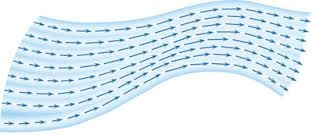### Steady Flow (Stream Line Flow)

It is the flow in which the velocity of fluid particles crossing a particular point is the same at all the times. Thus, each particle takes the same path as taken by a previous particle through the point.

### Steady, Streamline or Laminar FlowThe flow of fluid is said to be streamline if the velocity at any point in the fluid remains constant with time (in magnitude as well as in direction) in this case energy needed to drive the fluid is used up in overcoming the viscous force between its layers. All particles passing through a point in a steady flow follow the same path. The paths are known as streamlines> An example is water slowly flowing through a pipe.

### Turbulent Flow

When the motion of a particle varies rapidly in magnitude and direction, the flow is said to be turbulent. In other words, when the velocity exceeds beyond the critical velocity, the paths and velocities of liquid change continuously and haphazardly. This flow is called turbulent flow. An example is water coming out of a fountain.

### Critical Velocity

If in the case of a steady flow of fluids the velocity of flow is gradually increased it is found that the motion remains steady (streamline or laminar) upto a certain limit. If the velocity of flow crosses a certain limit the fluid particles do not follow the path of the preceding one and the motion becomes turbulent. The maximum velocity upto which fluid motion remains steady is called critical velocity. According to Reynold, in case of motion of fluids in narrow tubes, critical velocity depends on the density r and coefficient of viscosity η of the fluid as well as radius of the tube. i.e.

Vc  = η/rρ     or   Vc = R η/ρr

Here R is a dimensionless constant called Reynold’s number. For steady flow R < 2000. For 2000<R<3000, flow is transitional and for R>3000 flow is turbulent.

Pause: We shall be studying about viscosity later on in the course of this chapter.

Note: In further discussions, we shall be considering only the streamline motion of a non-viscous incompressible fluid.

Enquiry: Will the velocity of a fluid remain constant even if the tube through which it flows has constrictions?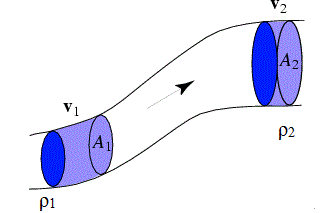In case of a fluid flowing through a tube of non-uniform cross-section, the product of the area of cross-section, density and the velocity of flow is same at every point in the tube. This is known as the principle of continuity, which actually means that amount of mass remains constant. For a fluid, mass flowing in through end B=mass flowing out from C. If the fluid is compressible

A1V1ρ1 = A2V2ρ2

If the fluid is incompressible then ρ1 = ρ2.

Therefore, A1V1 = A2V2 = Constant

Therefore, we can infer that if the cross-sectional area of a tube changes, the velocity of flow will also change.

### Illustration

Let us analyze the problem above. Why does the fluid flow through the hole? We know that fluid exerts, force on the walls of container and walls of container produce equal and opposite reaction. But at the hole there is no wall to produce a reaction. Hence the volume element near the hole experiences a net downward force and accelerates out of the hole. In terms of pressure, we may say that pressure inside the container (near the hole) is greater than pressure outside the container and fluid flows towards lower pressure. Also observe that in the container, velocity of an infinitesimal volume element is less than its velocity when it emerges out.

Similarly, consider a waterfall. Water gains speed as it reaches the bottom. Let us think why it falls down. The answer is its tendency to reduce its potential energy. Its potential energy gets reduced but kinetic energy is increased. Note that pressure was same at both ends i.e. atmospheric pressure. (This can also be explained by Newton’s laws of motion)

### Line of Flow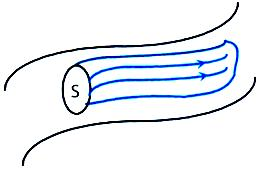It is the path taken by a particle in flowing liquid. In case of a steady flow, it is called streamline. Consider an area S in a fluid in steady flow. Draw streamlines from all the points on the periphery of S. These streamlines enclose a tube, on which S in a cross-section.

No fluid enters or leaves across the surface of this tube.

### Equation of Continuity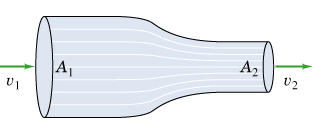In a time Dt, the volume of liquid entering the tube of flow in a steady flow is (A1v1 Δt). The same volume must flow out as the liquid is incompressible. The volume flowing out in time Δt is A2 v2 Δt.

=> A1 v1 – A2 v2.

Mass flow rate = ρAV (where ρ is the density of the liquid).

### Stokes LawWhen a body moves through a fluid, the fluid in contact with the body is dragged with it. This establishes relative motion in fluid layers near the body, due to which viscous starts operating. The fluid exerts viscous force on the body to oppose its motion. The magnitude of the viscous force depends on the shape and size of the body, its speed and viscosity of the fluid. As the viscous force depends on velocity of the body it keeps on increasing with the increase in velocity. If it balances the net downward force then body falling freely in a viscous medium attains a constant velocity, which is called terminal velocity. If a shape of radius r moves with a velocity v through a fluid of viscosity η, the viscous force opposing the motion of the sphere is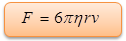This law is called Stokes law. The sphere dropped in the fluid moves down with a decreasing acceleration until the net force on it becomes zero. At this point it is said to have attained terminal velocity.

Viscous force = Weight – Upthrust

or,  F = 6pηrvT. = (4/3 πr3 )ρg – (4/3 πr3 σ)g.

Where r is the density of the solid and σ is the density of the fluid.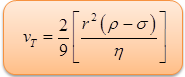Stokes Law states that the speed or velocity with which particles settle out of a liquid medium is dependent on a constant factor and the radius of the particles. The bigger the particle, the faster it will fall out of suspension.

Enquiry: Can we study fluids in motion?

Motions of smoke from chimney, flow of water in river, flow of any gas in a tube are examples of flow of fluids. This is generally a complex stream of mechanics. But, if we restrict ourselves to a simpler type of motion, i.e. steady, streamline or laminar flow and not turbulent flow then we can study the behavior of such fluids.

A cubical block of wood of specific gravity 0.5 and chunk of concrete of specific gravity 2.5 are fastened together. The ratio of the mass of the wood to the mass of concrete, which makes the combination to float with its entire volume submerged under water is (0.x). Find value of x.

### Solution

W = Upthrust

Or (m1+m2)g = [(m1/0.5 ρw) + (m2/2.5 ρw)]ρwg

Or m1+m2 = 2m1 + 0.4 m2

Thus, m1/m2 = 0.6 = 3/5

##• The velocity of any molecule, at a point, is independent of time.
• The layer of liquid in contact with the solid surface is at rest.
• The liquid in streamlined flow can be supposed to be in the form of parallel layers one above the other.
• Velocity of flow of liquid varies inversely as the area of cross-section of the opening from where the liquid comes out.
• In laminar flow, the velocities of molecules lying on different stream lines may be different.Question 1

Deep water almost runs still when surface water flows in rivers. What does it explain?

(a) Magnus effect          (b) Equation of continuity

(c) Surface energy         (d)  Bernoulli’s equation

Question 2

The rain drop falling from the sky neither hit us nor make holes on the ground because they move with:

(a) constant acceleration      (b) variable acceleration

(c) variable speed                (d) constant terminal velocity

Question 3

Ball pen functions on the principle of:

(a) Viscosity                (b) Boyle’s law

(c) Gravitational force    (d) Surface tension

Question 4

When the velocity of a liquid flowing steadily in a tube increases, its pressure:

(a) Decreases                 (b) Increases

(c) Remains the same    (d) Is zeroQ.1 Q.2 Q.3 Q.4 d d a a

### Related Resources• Complete Physics Course – Class 12
• OFFERED PRICE: Rs. 2,756

### Course Features

• 101 Video Lectures
• Revision Notes
• Previous Year Papers
• Mind Map
• Study Planner
• NCERT Solutions
• Discussion Forum
• Test paper with Video Solution